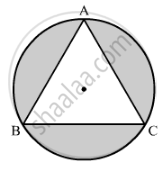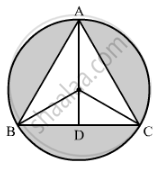Advertisement Remove all ads

# On a Circular Table Cover of Radius 42 Cm, a Design is Formed by a Girl Leaving an Equilateral Triangle Abc in the Middle, as Shown in the Figure. Find the Covered Area of the Design.V - Mathematics

Sum

On a circular table cover of radius 42 cm, a design is formed by a girl leaving an equilateral triangle ABC in the middle, as shown in the figure. Find the covered area of the design. ["Use" sqrt(3) = 1.73, pi =22/7]Advertisement Remove all ads

#### Solution

Construction:  Join AO and extend it to D on BC.Radius of the circle, r = 42 cm
∠OCD= 30°

cos30° = "DC"/"OC"

=> sqrt(3)/2 = "DC"/42

⇒ "DC" = 21sqrt(3)

=> "DC" = 2xx"DC" = 42sqrt(3) = 72.66  "cm"

sin 30°="OD"/"OC"

=>  1/2="OD"/42

⇒ OD = 21 cm

Now, AD = AO + OD = 42 + 21  = 63 cm

Area of shaded region =  Area of circlec - Area of triangle ABC

= pi(OA)^2-1/2xx"AD"xx"AB"

=22/7(42)^2-1/2xx63xx72.66

= 5544 - 2288.79

= 3255.21 cm

Is there an error in this question or solution?
Advertisement Remove all ads

#### APPEARS IN

RS Aggarwal Secondary School Class 10 Maths
Chapter 18 Area of Circle, Sector and Segment
Exercise 18B | Q 48 | Page 835
Advertisement Remove all ads

#### Video TutorialsVIEW ALL 

Advertisement Remove all ads
Share
Notifications

View all notifications

Forgot password?
Course